# coinheven's profile

## General stats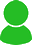3 followers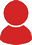1 following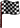22449 pts - Legend[?] - 72nd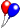7038 pts - Racer[?] - 187th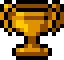99 pts - 2 challenges won - 141st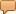21 messages -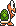Koopa[?]
39 created circuits - 9 cups
35 challenges created
0 shared character
0 record in time trial
4 topics followed

United States
Registered since 14/03/2019
Last connection: 20/06/2019

## Last messages on the forum :21 -Koopa22449 pts ★ Legend7038 pts ★ Racer
United States
I still don't know why im here. I have better things to do.21 -Koopa22449 pts ★ Legend7038 pts ★ Racer
United States
easly the gamecube one.21 -Koopa22449 pts ★ Legend7038 pts ★ Racer
United States
Temmie wrote:
h   a   r   d       m   a   n       h   a   r   d       m   a   n       h   a   r   d       m   a   n       h   a   r   d       m   a   n       h   a   r   d       m   a   n       h   a   r   d       m   a   n       h   a   r   d       m   a   n       h   a   r   d       m   a   n       h   a   r   d       m   a   n       h   a   r   d       m   a   n       h   a   r   d       m   a   n       h   a   r   d       m   a   n       h   a   r   d       m   a   n       h   a   r   d       m   a   n       h   a   r   d       m   a   n       h   a   r   d       m   a   n       h   a   r   d       m   a   n       h   a   r   d       m   a   n       h   a   r   d       m   a   n       h   a   r   d       m   a   n       h   a   r   d       m   a   n       h   a   r   d       m   a   n       h   a   r   d       m   a   n       h   a   r   d       m   a   n       h   a   r   d       m   a   n       h   a   r   d       m   a   n       h   a   r   d       m   a   n       h   a   r   d       m   a   n       h   a   r   d       m   a   n       h   a   r   d       m   a   n       h   a   r   d       m   a   n       h   a   r   d       m   a   n       h   a   r   d       m   a   n       h   a   r   d       m   a   n       h   a   r   d       m   a   n       h   a   r   d       m   a   n       h   a   r   d       m   a   n       h   a   r   d       m   a   n       h   a   r   d       m   a   n       h   a   r   d       m   a   n       h   a   r   d       m   a   n       h   a   r   d       m   a   n       h   a   r   d       m   a   n

yeah

Gemini Man is better

## Best created circuits :

 Pipe Maze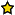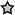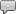3Peach Roadway1Cotton Kingdom 10Sandy Ruins3Half-8-Roadway0